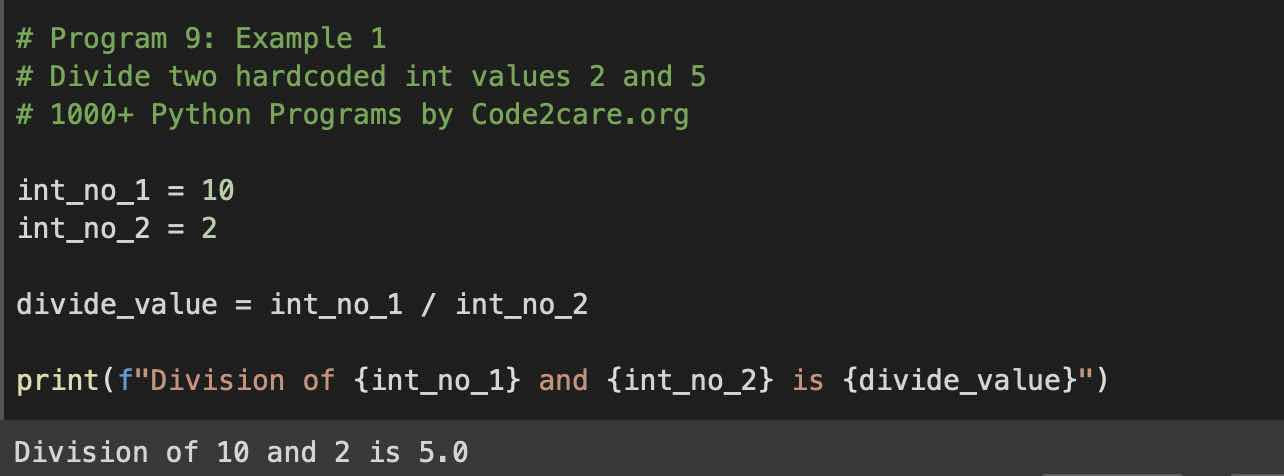# Program 9: Divide Two Numbers - 1000+ Python Programs

Question 8)

Write a program in Python to divide two numbers.
Write a program in Python to divide numbers 10 by 2.
Write a program to divide two numbers by taking user input using Python Programming.
Program to find the divide two integer values in Python.
Program to find the divide two float values in Python.

## Code Example 1: Divide two Integers

``````# Program 9: Example 1
# Divide two hardcoded int values 2 and 5
# 1000+ Python Programs by Code2care.org

int_no_1 = 10
int_no_2 = 2

divide_value = int_no_1 / int_no_2

print(f"Division of {int_no_1} and {int_no_2} is {divide_value}")``````
Output:

Division of 10 and 2 is 5## Code Example 2: Divide two integer numbers from user input

``````# Program 9: Example 2
# Divide two ints from the user input
# 1000+ Python Programs by Code2care.org

int_no_1 = int(input("Enter int 1: "))
int_no_2 = int(input("Enter int 2: "))

divide_value = int_no_1 / int_no_2

print(f"Division of {int_no_1} by {int_no_2} is {divide_value}")``````
Output:

Enter int 1: 20
Enter int 2: 2
Division of 20 by 2 is 10

## Code Example 3: Divide two floats numbers from user input

``````# floats 8: Example 3
# Divide two ints from the user input
# 1000+ Python Programs by Code2care.org

float_no_1 = float(input("Enter int 1: "))
float_no_2 = float(input("Enter int 2: "))

divide_value = float_no_1 / float_no_2

print(f"Division of {int_no_1} by {int_no_2} is {divide_value}")``````
Output:

Enter int 1: 10.4
Enter int 2: 2.4
Division of 10.4 and 2.4 is 24.96

## Explaination:

List of functions used,

1. print(): Built-in function to print a string on the console with f-strings formatting.
2. float(): Built-in function to convert String argument to float value.
3. input(): Built-in function to accept user input

### Steps:

1. Create a variable to hold the first integer/float
2. Create a variable to hold a second integer/float
3. Create a third variable to hold the division of two variables using the / (slash) division operator.
4. Print the result using print() function.
-

Have Questions? Post them here!